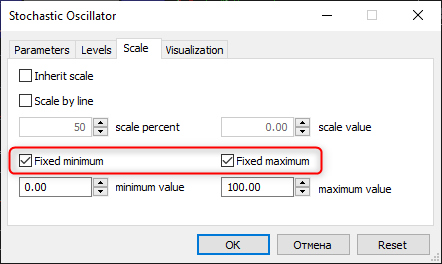# MetaTrader 5 build 3210: New matrix methods and control over indicator minimum/maximum values

## Revised Sharpe Ratio calculation algorithm to match the traditional formula, in which the value corresponds to a one-year interval

11 February 2022

### MQL5

1. Added Min, Max, ArgMin, ArgMax and Sum functions for vectors and matrices. Use the functions to find the minimum and the maximum values, relevant indexes and the sum.
2. Added support for Flat methods for the matrix. With these methods, a matrix element can be addressed through one index instead of two.
```double matrix::Flat(ulong index) const;      // getter
void matrix::Flat(ulong index,double value); // setter```

Pseudocode for calculating the address of a matrix element:

```ulong row=index / mat.Cols();
ulong col=index % mat.Cols();

mat[row,col]```

For example, for 'matrix mat(3,3)', access to elements can be written as follows:

reading: 'x=mat.Flat(4)', which is equivalent to 'x=mat'
writing: 'mat.Flat(5, 42)', equivalent to 'mat=42'

If the function is called with an invalid matrix index, the OutOfRange critical execution error will be thrown.

3. Improved formatting of floating-point numbers in MQL5-program input parameters. When reading some real numbers, numbers with many zeros were substituted into the input parameters, for example, 0.4 was represented as 0.400000000002.
4. Fixed errors in the Math\Stat\Math.mqh math library. The MathSample function from this library has been revised to match the traditional behavior of similar math libraries when sampling with backtracking.
5. Fixed CopyTicks/CopyTicksRange error which could cause the return of outdated data when crossing over the midnight, when no ticks are provided for the financial instrument.
6. Added new INDICATOR_FIXED_MINIMUM and INDICATOR_FIXED_MAXIMUM values into the ENUM_CUSTOMIND_PROPERTY_INTEGER enumeration.
Using these properties, you can fix or unfix the minimum and maximum indicator values using the IndicatorSetInteger function. When calling IndicatorSetInteger(INDICATOR_FIXED_MINIMUM/INDICATOR_FIXED_MAXIMUM, true), the current minimum or maximum value is used.### Tester

1. Revised Sharpe Ratio calculation algorithm to match the traditional formula, in which the value corresponds to a one-year interval. The previous algorithm was based on the variability of obtained PnL and it ignored equity fluctuations against open positions. Now the calculation includes equity movements, while the Sharpe ratio is interpreted in a classical way:
• Sharpe Ratio < 0              The strategy is unprofitable and is not suitable. Bad.
• 0 < Sharpe Ratio  < 1.0    The risk does not pay off. Such strategies can be considered when there are no alternatives. Indefinite.
• Sharpe Ratio ≥ 1.0          If the Sharpe ration is greater than one. This can mean that the risk pays off and that the portfolio/strategy can show results. Good.
• Sharpe Ratio ≥ 3.0          A high value indicates that the probability of obtaining a loss in each particular deal is very low. Very good.

### Terminal

1. Optimized memory consumption by the terminal.
2. Improved platform operation with a network subsystem to enhance performance and to reduce network delays.
3. Removed the display of the zero grid level in indicators when grid rendering is disabled.